Type
Quiz
Book Title
Fundamentals of Corporate Finance Standard Edition 9th Edition
ISBN 13
978-0073382395

### 978-0073382395 Chapter 7 Questions and Problems 14-24

April 3, 2019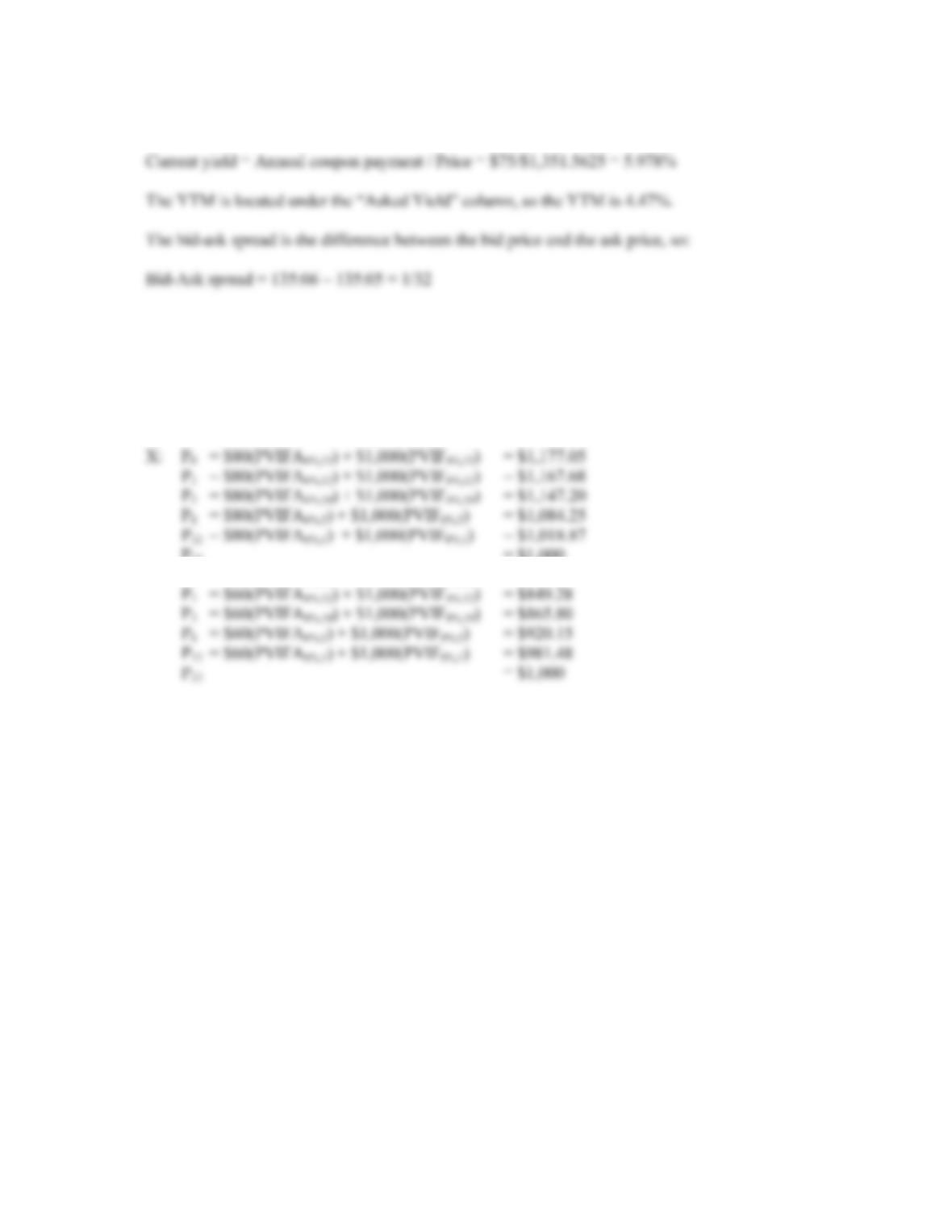CHAPTER 7 B-123
14. This is a premium bond because it sells for more than 100% of face value. The current yield is:
Intermediate
15. Here we are finding the YTM of semiannual coupon bonds for various maturity lengths. The bond
price equation is:
P = C(PVIFAR%,t) + \$1,000(PVIFR%,t)
P
13 = \$1,000
Y: P0 = \$60(PVIFA8%,13) + \$1,000(PVIF8%,13) = \$841.92
P
All else held equal, the premium over par value for a premium bond declines as maturity approaches,
and the discount from par value for a discount bond declines as maturity approaches. This is called
“pull to par.” In both cases, the largest percentage price changes occur at the shortest maturity
lengths.
Also, notice that the price of each bond when no time is left to maturity is the par value, even though
the purchaser would receive the par value plus the coupon payment immediately. This is because we
calculate the clean price of the bond.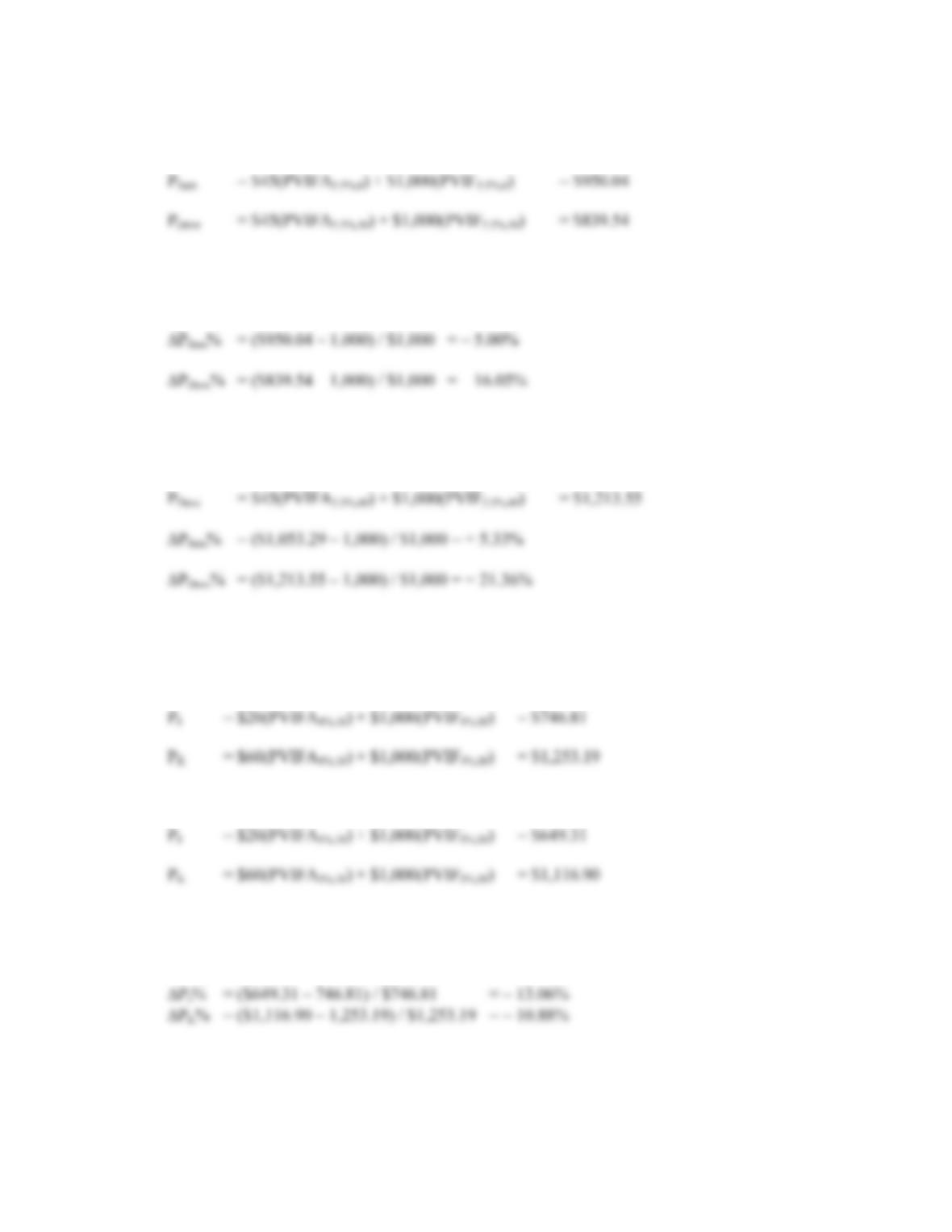B-124 SOLUTIONS
16. Any bond that sells at par has a YTM equal to the coupon rate. Both bonds sell at par, so the initial
YTM on both bonds is the coupon rate, 9 percent. If the YTM suddenly rises to 11 percent:
P
The percentage change in price is calculated as:
Percentage change in price = (New price – Original price) / Original price
If the YTM suddenly falls to 7 percent:
P
Sam = \$45(PVIFA3.5%,6) + \$1,000(PVIF3.5%,6) = \$1,053.29
P
All else the same, the longer the maturity of a bond, the greater is its price sensitivity to changes in
interest rates.
17. Initially, at a YTM of 8 percent, the prices of the two bonds are:
P
If the YTM rises from 8 percent to 10 percent:
P
The percentage change in price is calculated as:
Percentage change in price = (New price – Original price) / Original price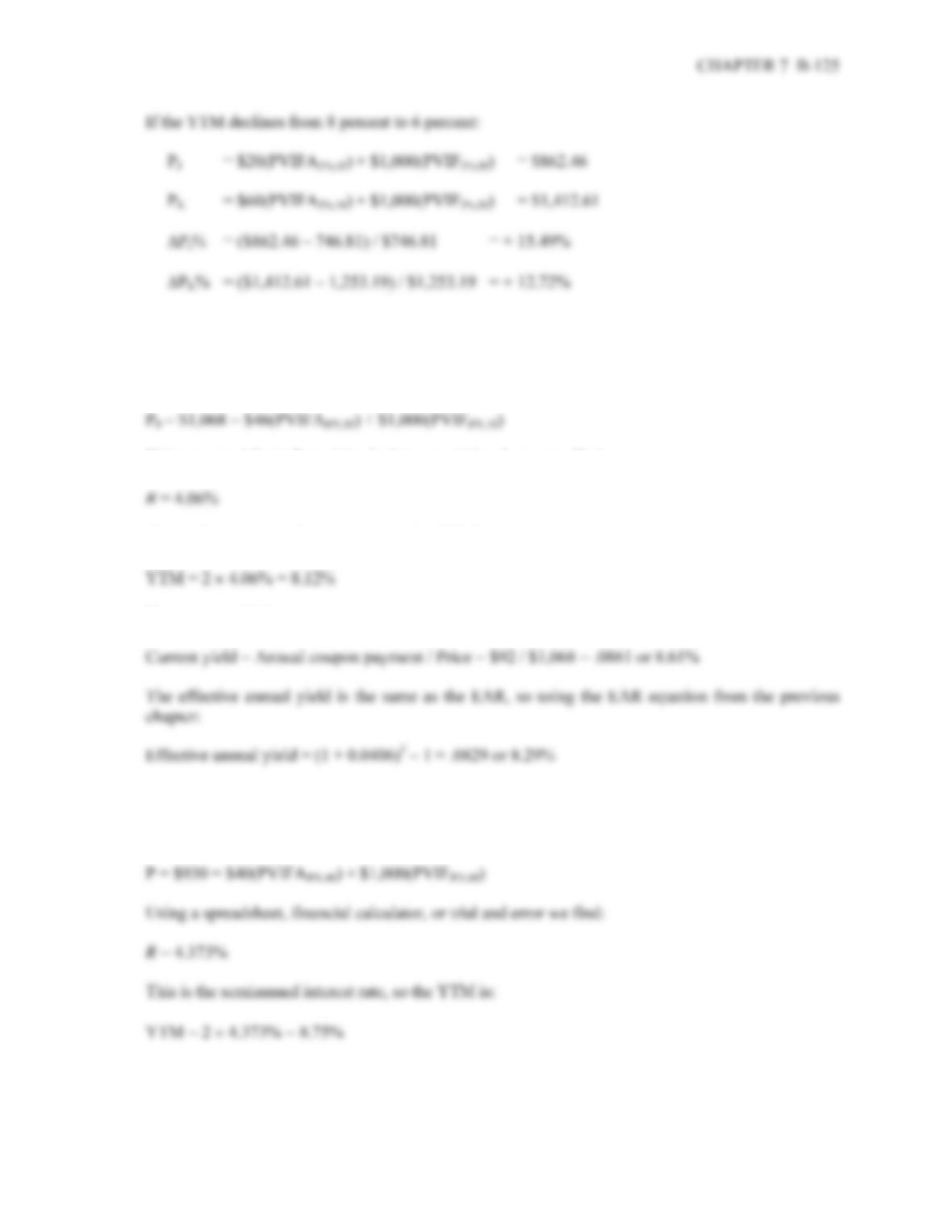All else the same, the lower the coupon rate on a bond, the greater is its price sensitivity to changes
in interest rates.
18. The bond price equation for this bond is:
P
Using a spreadsheet, financial calculator, or trial and error we find:
This is the semiannual interest rate, so the YTM is:
The current yield is:
19. The company should set the coupon rate on its new bonds equal to the required return. The required
return can be observed in the market by finding the YTM on outstanding bonds of the company. So,
the YTM on the bonds currently sold in the market is: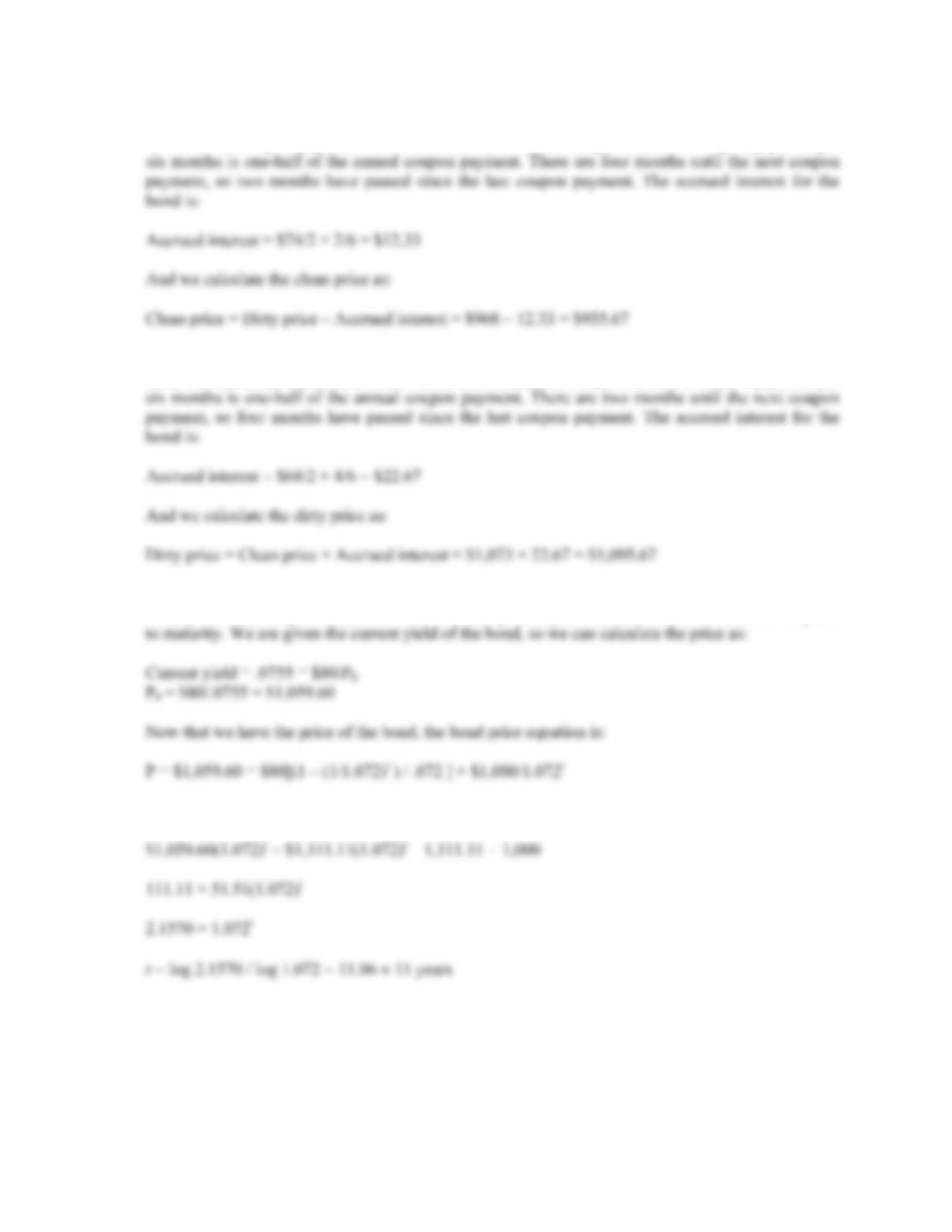B-126 SOLUTIONS
20. Accrued interest is the coupon payment for the period times the fraction of the period that has passed
since the last coupon payment. Since we have a semiannual coupon bond, the coupon payment per
21. Accrued interest is the coupon payment for the period times the fraction of the period that has passed
since the last coupon payment. Since we have a semiannual coupon bond, the coupon payment per
22. To find the number of years to maturity for the bond, we need to find the price of the bond. Since we
already have the coupon rate, we can use the bond price equation, and solve for the number of years
We can solve this equation for t as follows:
The bond has 11 years to maturity.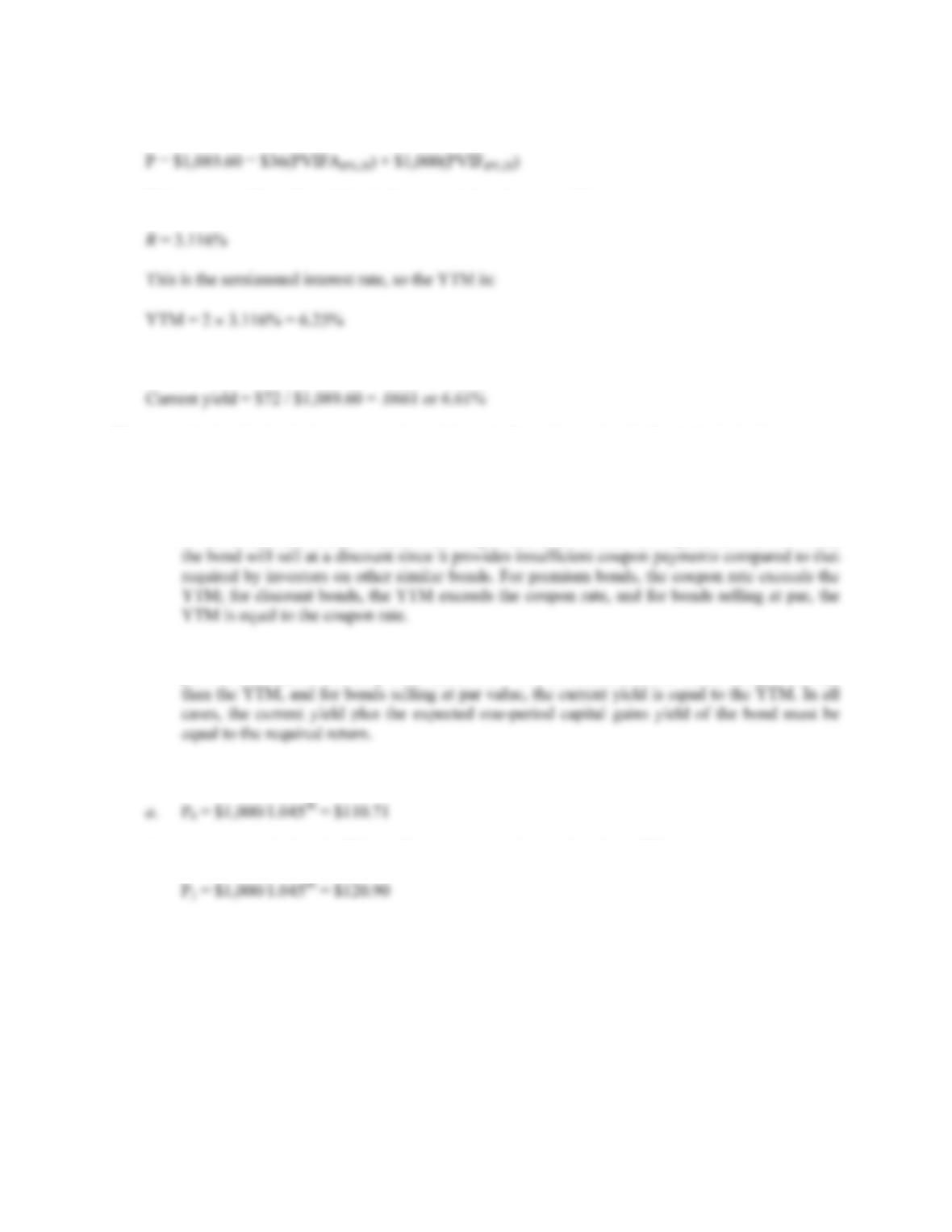CHAPTER 7 B-127
23. The bond has 14 years to maturity, so the bond price equation is:
Using a spreadsheet, financial calculator, or trial and error we find:
The current yield is the annual coupon payment divided by the bond price, so:
24. a. The bond price is the present value of the cash flows from a bond. The YTM is the interest rate
used in valuing the cash flows from a bond.
b. If the coupon rate is higher than the required return on a bond, the bond will sell at a premium,
since it provides periodic income in the form of coupon payments in excess of that required by
investors on other similar bonds. If the coupon rate is lower than the required return on a bond,
c. Current yield is defined as the annual coupon payment divided by the current bond price. For
premium bonds, the current yield exceeds the YTM, for discount bonds the current yield is less
25. The price of a zero coupon bond is the PV of the par, so:
a. P0 = \$1,000/1.04550 = \$110.71
b. In one year, the bond will have 24 years to maturity, so the price will be:
P